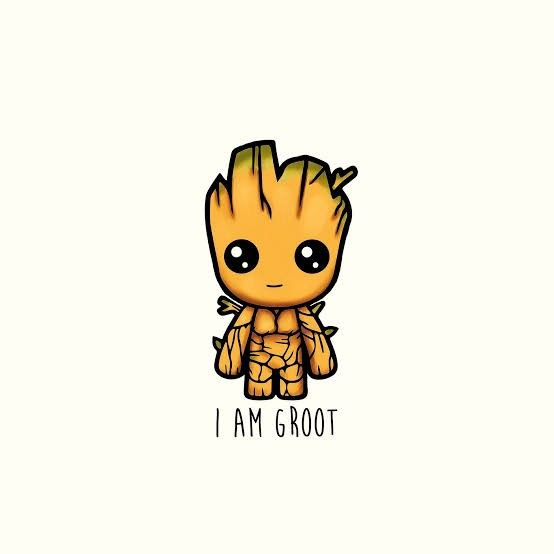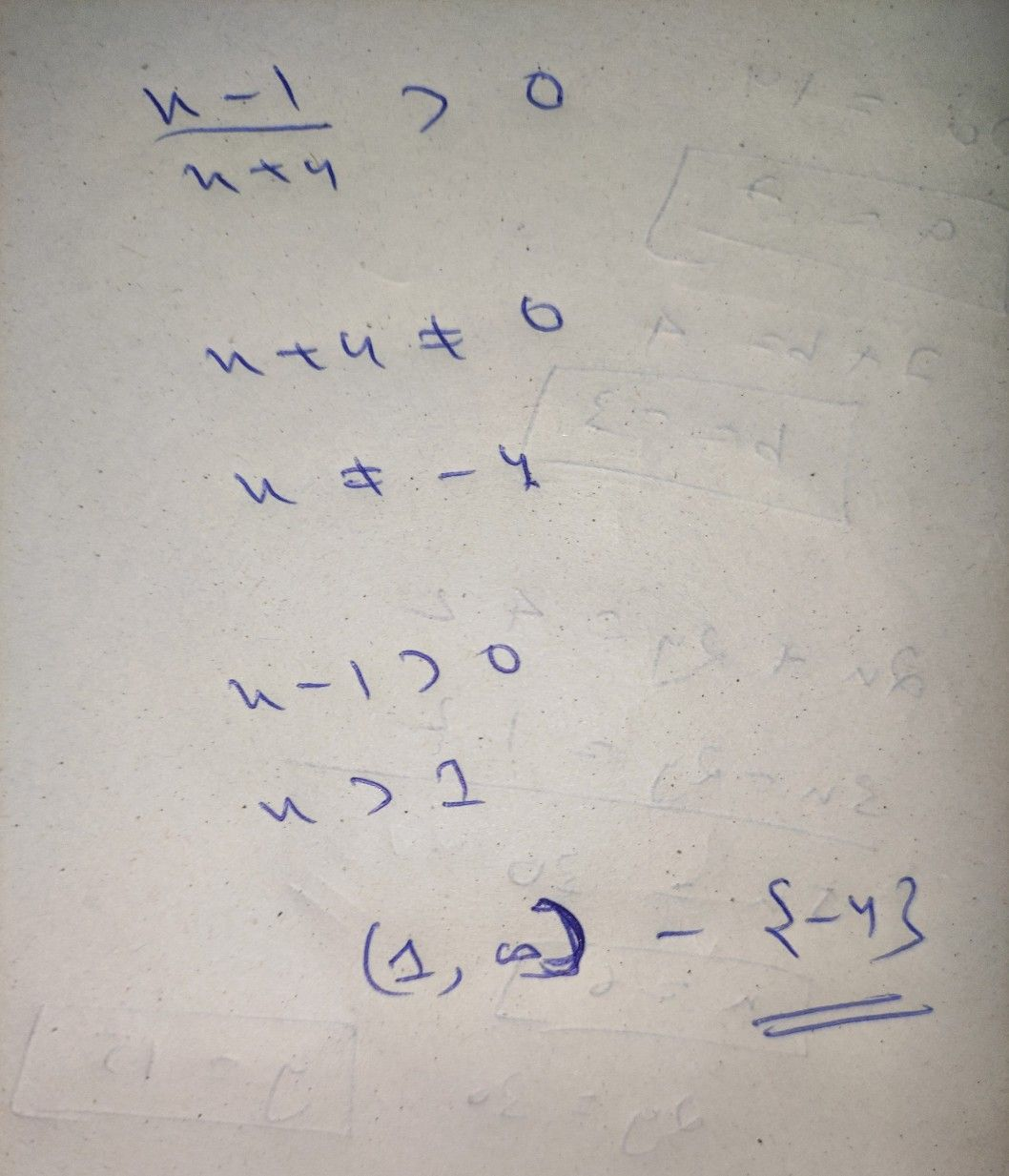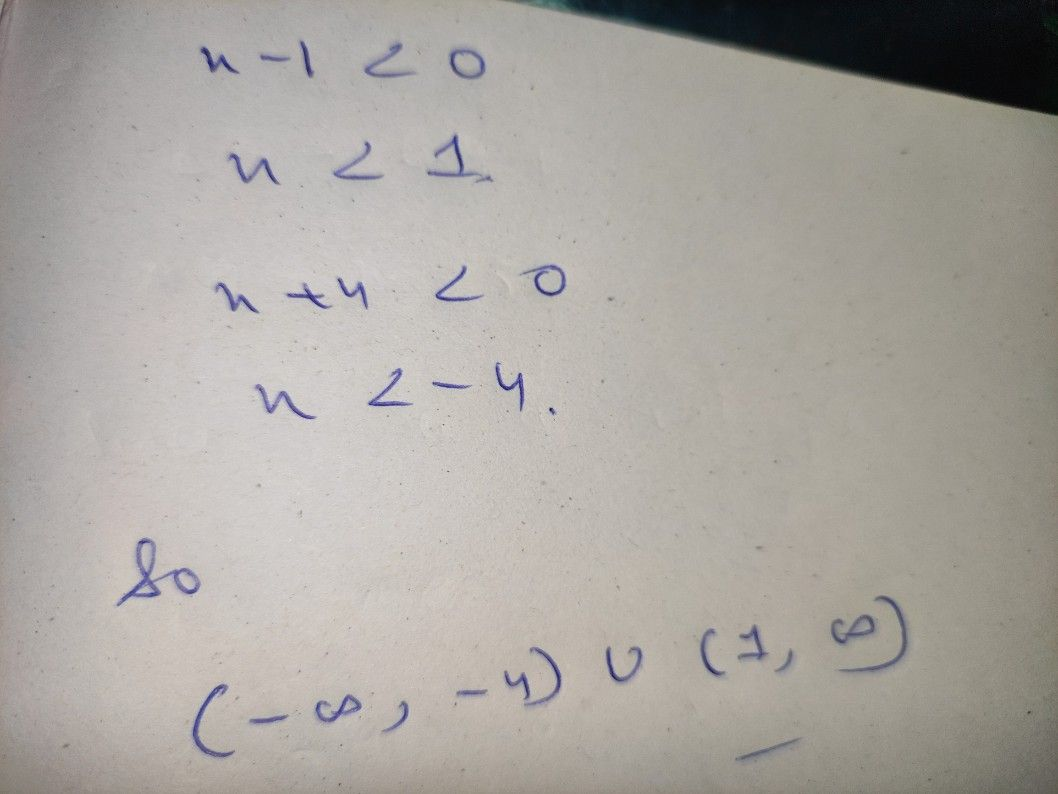Symbol
Problem$ucion$ Choose the letter of the correct answer. What is the solution set of the rational inequality $\dfrac {x-1} {x+4}>07$ $3$ $\left(-\infty$ $4\right)11\left(1$ $m\right)$
7th-9th grade
Other
Search count: 107
SolutionQanda teacher - AnweshaStudent
thank you mam